# Total Number of Possible Binary Search Trees with n Keys

Key takeaway: An excellent problem to learn the idea of catalan number and problem solving using dynamic programming.

### Problem understanding

Write a program to find the number of structurally unique binary search trees (BSTs) that have exactly n nodes, where each node has a unique integer key ranging from 1 to n. In other words, we need to determine the count of all possible BSTs that can be formed using n distinct keys.

#### Examples

Input: n = 1, Output: 1

Input: n = 2, Output: 2

Input: n = 3, Output: 5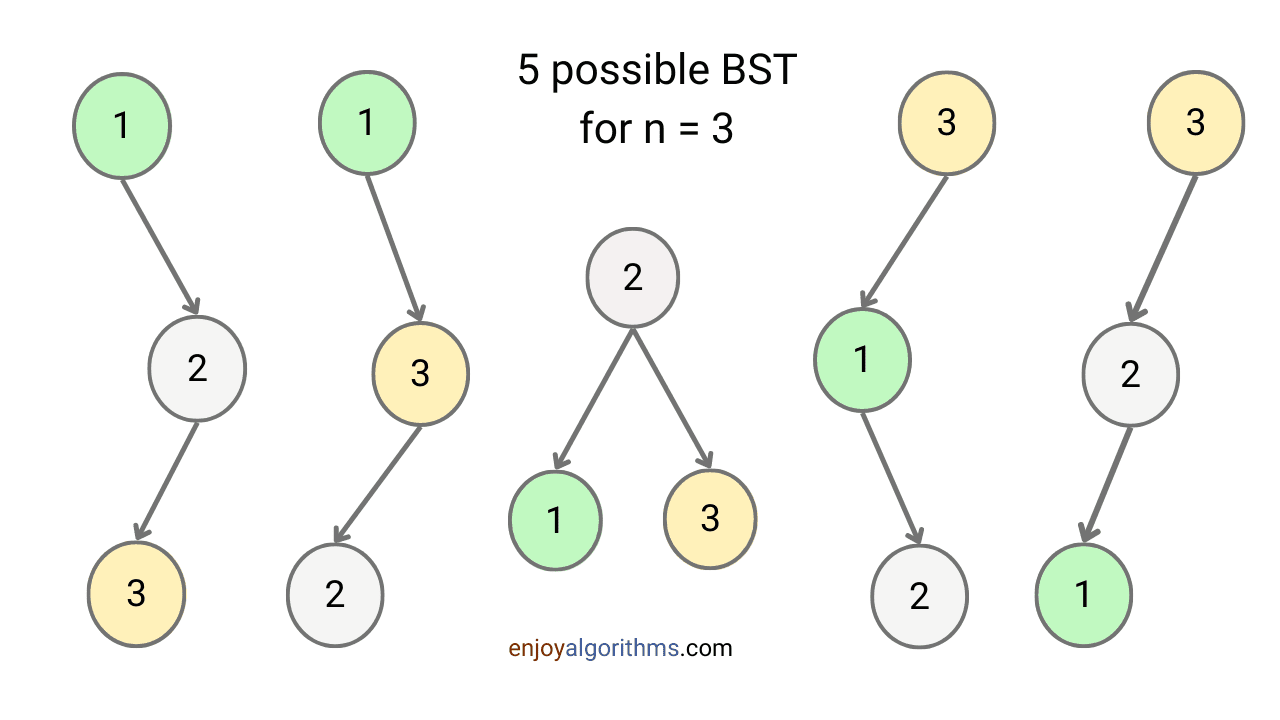### Discussed solution approaches

• Brute force solution using recursion
• Solution idea using top down memoization of DP
• Solution idea using bottom up approach of DP
• Solution idea using the binomial coefficient value of nth Catalan number.

### Brute force solution using recursion

#### Solution idea and steps

A binary search tree follows the BST property, where relative order between elements is important. So without loss of generality, let’s assume C(n) is the count of the total number of BSTs with n nodes.

If we consider all possible BSTs, then all n keys are candidates for the root node. For each choice of the root node, n -1 non-root nodes must be divided into two sets: 1) Nodes with keys smaller than the key of the root node 2) Nodes with keys greater than the key of the root node.

Suppose we choose the node with ith key as the root node. Then i - 1 nodes are smaller than the root node, and n - i nodes are larger than the root node. Now the problem of counting BSTs with the ith node as the root node is divided into two subproblems:

• The total count of different BSTs for the left subtree with i -1 different keys, which is C(i - 1).
• The total count of different BSTs for the right subtree with n - i different keys, which is C(n - i).

These arrangements in the left and right subtrees are independent of each other, so we can multiply these two terms together to get the total count of BSTs with ith node as the root node. So the total count of BSTs with the ith node as the root node = (Total BST count for the left subtree) * (Total BST count for the right subtree) = C(i -1) * C(n - i).

Since we have n different choices for the root node, we can sum over i = 1 to n to obtain the total count of binary search trees with n nodes. C(n) = Σ(i = 1 to n) C(i -1) * C(n - i) = Σ(i = 0 to n - 1) C(i) * C(n - 1 - i). This is expression for the nth Catalan number. Hint: Compare this equation with the recurrence relation written in the following image.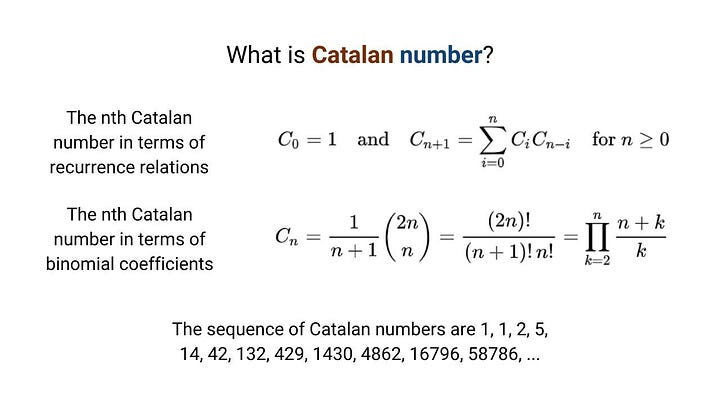From the above observation, we can conclude that: To count all possible binary search trees with n nodes, we need to find the value of nth Catalan number. So one solution idea is to write recursive code to solve the recurrence relation of the nth Catalan number.

Recursive structure

countUniqueBST(n) = Σ(i = 0 to n-1) countUniqueBST(i) * countUniqueBST(n - i - 1).

Base case

Here we have two base cases: C(0) = 1 and C(1) = 1. The idea is simple: There is only one way to make a BST with 1 or 0 node.

#### Solution code C++

``````unsigned long int countUniqueBST(int n)
{
if (n == 1 || n == 0)
return 1;

unsigned long int countLeft, countRight, count = 0;
for (int i = 0; i < n; i = i + 1)
{
countLeft = countUniqueBST(i);
countRight = countUniqueBST(n - 1 - i);
count = count + countLeft * countRight;
}
return count;
}``````

#### Solution analysis

Suppose T(n) is the time complexity function for input size n. The recurrence relation to calculate time complexity is: T(n) = Σ(i = 0 to n - 1) T(i) * T(n - i -1).

As discussed above, this equation is similar to the recurrence relation of nth Catalan number. So the value of T(n) will be the value of the nth Catalan number, which is exponential. Note: The value of the nth Catalan number in power of n grows roughly as 4^n / (pi * n). For more understanding, you can follow wikipedia article on catalan number.

So the time complexity of the above recursive code is exponential time. This is highly inefficient because recursion is solving same subproblems again and again. How? Let’s think! For input size n, we are using subproblems of input size 0, 1, 2, …, n-2, and n-1. Similarly, for solving input size n-1, we are again using subproblems of input size 0, 1, 2, …, n-3, and n-2. And so on!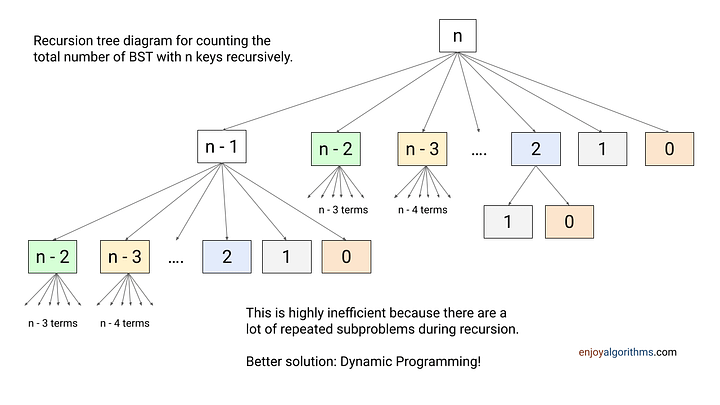We can observe repeated subproblems in the above diagram. If we expand the recursion tree diagram further, there will be a lot of repeated subproblems. So what would be an efficient solution? Since there are overlapping subproblems, we can use the idea of dynamic programming to solve this.

### Using top-down approach of dynamic programming

In the top-down (memoization) approach, we solve the problem recursively by breaking it down into smaller sub-problems. But instead of solving the subproblems again and again during recursion, we store solution of the subproblems in a cache or memoization table so that we can reuse them later when we need them.

#### Solution steps

Suppose function countUniqueBST takes an integer n as input and returns the number of unique BSTs that can be formed with n nodes.

• Base case: If n is 0 or 1, function returns 1, as there is only one possible BST that can be formed with 0 or 1 nodes.
• To memoize the results of subproblems, we use the count[n + 1] array to store the solution for every possible subproblem. Note that in the recursive solution, there are n + 1 unique subproblems of input size 0, 1, 2, ..., n - 1, and n. These subproblems will be repeated during recursion, and we need to take a table size of n + 1 to store their values. For example, we will store the subproblem solution of input size i at index i of the table.
• Before starting the recursive process, we initialize all the values of this array to -1 to indicate that the result has not yet been computed.
• Now during recursion, if the value of count[i] is not -1, we have already computed the number of unique BST for input size i. So we return this precomputed value count[i] as the output.
• If count[i] is -1, we have not computed the number of unique BST for input size i. So we recursively calculate it by iterating through all possible root nodes j (where j ranges from 1 to i). After this, we memoize the result by storing it in count[i] so that it can be retrieved directly if countUniqueBST is called again with the same value of i.

#### Solution code C++

``````// memoization table
vector<int> count;

unsigned long int countUniqueBST(int i)
{
// base case
if (i == 0 || i == 1)
return 1;

if (count[i] != -1)
return count[i];

unsigned long int res = 0;
for (int j = 1; j <= i; j = j + 1)
res = res + countUniqueBST(j - 1) * countUniqueBST(i - j);

// memoizing the result
count[i] = res;
return res;
}

int main()
{
int n;
cout << "Enter the value of n: ";
cin >> n;
// initializing memoization table with -1
count.assign(n + 1, -1);

// calling countUniqueBST function
unsigned long int result = countUniqueBST(n);

// printing the result
cout << "The number of unique BSTs with " << n << " nodes is " << result << endl;
return 0;
}``````

#### Time and space complexity analysis

Above approach will significantly improve the efficiency, because we are avoiding redundant calculations of repeated sub-problems. So what would be the time complexity?

We can compute the time complexity of countUniqueBST function by considering the number of unique sub-problems we need to solve. Since we are computing the result for each sub-problem only once and storing it in the memoization table, we will have to solve at most n sub-problems, where n is the input size. For each sub-problem, we need to iterate through all possible root nodes, which takes O(n) time. So the time complexity of countUniqueBST function using memoization is O(n²).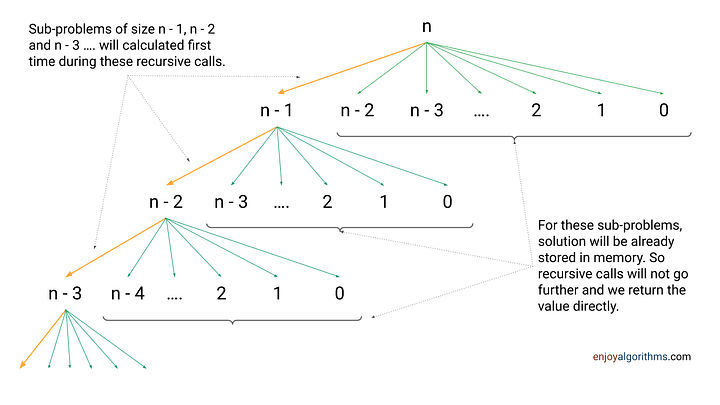Let’s understand this from another perspective! If we observe above recursion tree diagram, we will be calling sub-problem of size (n -1) one time, sub-problem of size (n -2) two times, sub-problem of size (n -3) three times, and so on.

Total number of recursive calls = 1 + 2 + 3 + …+ n - 1 + n = n (n + 1)/2 = O(n²). So time complexity = O(n²) * O(1) = O(n²). This is much better than brute force recursive approach.

Space complexity = Space complexity of extra memory + Space complexity of recursion call stack = O(n) + O(n) = O(n). Here size of recursion call stack will depend on the depth of the recursion tree, which is O(n). Think!

### Using Top-down approach of Dynamic Programming

#### Solution idea

In the bottom-up approach, we first solve smaller subproblems and then use the results of those subproblems to build the final solution. In other words, we start with the smallest subproblem and iteratively calculate the solution for larger subproblems until we arrive at the final solution.

• Table size and structure definition: There are n + 1 unique subproblems involved in the solution. So, we need a 1D array of size n + 1 to store solutions of subproblems in a bottom-up manner.
• Table initialization: Before storing solutions in a bottom-up manner, we initialize table with the solution of the smallest version of the problem, i.e., for input size 0 and 1.
• Iterative structure to fill the table: Using the recursive structure of recursive solution, we define an iterative structure to fill the table. Here, by using the base case stored at index 0 and 1, we iteratively compute the number of unique BSTs that can be formed with 2 nodes, then 3 nodes, and so on, until we arrive at n nodes.

Recursive structure: countUniqueBST(n) = Σ(i = 0 to n-1) countUniqueBST(i) * countUniqueBST(n - i - 1)

Iterative structure: For counting number of BST for input size i, we need to use value stored in entires j = 0 to i -1 i.e. count[i] = Σ(j = 0 to i - 1) count[j] * count[i - j - 1].

Note: If we observe, we are doing just three modifications in the recursive structure to get iterative structure: Replace "countUniqueBST" with "count", replace n with i and replace i with j. Think!

Returning the final solution: By end of the loop, our final solution of total number of BST for n nodes will get stored at index n + 1. So we return count[n + 1] as an output.

#### Solution code C++

``````unsigned long int countUniqueBST(int n)
{
int count[n + 1];
count = 1;
count = 1;
for (int i = 2; i <= n; i = i + 1)
{
count[i] = 0;
for (int j = 0; j < i; j = j + 1)
count[i] = count[i] + count[j] * count[i - j - 1];
}
return count[n];
}``````

#### Time and space complexity analysis

We are using two nested loops to fill the table in a bottom-up manner. So the overall time complexity = Total count of nested loop iterations * O(1).

• Total count of loop iterations = 2 + 3 + … + n = n(n+1)/2 – 1 = O(n²).
• So the time complexity = O(n²)*O(1) = O(n²).

The space complexity is O(n) for the n + 1 size count array.

### Using the binomial coefficient value of nth Catalan number

As we have seen above, the total count of all possible binary search tree with n nodes is the value of nth catalan number, which is mathematically equal to (2nCn)/(n + 1).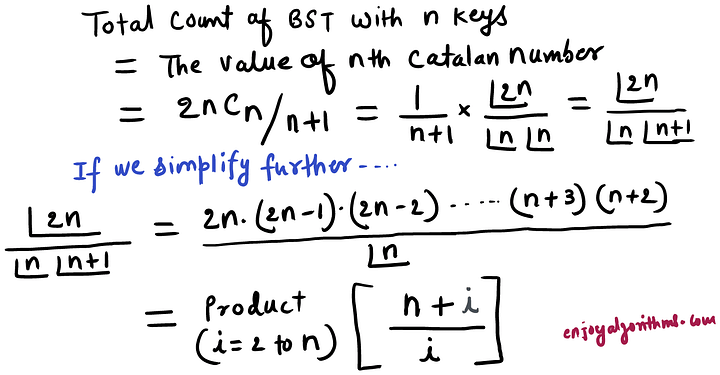As derived in the above image, one idea would be to find the simple product of (n + i)/i from i = 2 to n to get the count of all possible BST. This can be very simple to implement in O(n) time and O(1) space.

#### Solution code C++

``````unsigned long int countBSTUsingCatalan(int n)
{
unsigned long int bstCount = 1;
for (int i = 2; i <= n; i = i + 1)
bstCount = bstCount* (n + i);

for (int i = 2; i <= n; i = i + 1)
bstCount = bstCount/ i;

return bstCount;
}``````

#### Another version of implementation

Another way to find the total count of all possible BSTs is to modify the equation we derived earlier. The equation is: Total BST count = The value of nth Catalan number = 2nCn/(n + 1).

We can further simplify this equation by expanding 2nCn and rearranging the terms. We get: 2nCn/(n + 1) = (2n)!/(n! (n + 1)!) = (2n)(2n — 1)(2n — 2)…(n + 1)/n! 1/ (n + 1)) = [Product of (2n — i)/(i + 1) from i = 0 to n — 1] / (n + 1).

We can also implement this easily in O(n) time and O(1) space.

``````unsigned long int countBSTUsingCatalan(int n)
{
unsigned long int count = 1;
for (int i = 0; i < n; i++)
{
count = count * (2*n - i);
count = count/ (i + 1);
}

return count/(n+1);
}``````

#### Time and space complexity analysis

We are using single loop and performing constant operation at each iteration. So time complexity = O(n). We are using constant extra space. So space complexity = O(n).

### Critical ideas to think!

• What is the more efficient solution approach for finding the total count of all possible binary search trees with n nodes: top-down or bottom-up?
• What are some important uses of Catalan numbers in coding problem solving?
• Can we try to solve this problem using some other approach?
• What is the mathematical proof of the Catalan number?
• In the last approach, can we implement the solution idea using single loop? Is there some other way to implement this?
• Is this the correct code to count all possible Binary Search Trees with n nodes? If yes, then what is the idea behind this code?
``````unsigned long int countBSTUsingCatalan(int n)
{
unsigned long int bstCount = 1;
for (int i = 0; i < n; i = i + 1)
{
bstCount = bstCount * (4 * i + 2);
bstCount = bstCount/(i + 2);
}
return bstCount;
}``````

### Similar coding questions to practice

• Count total number of permutations of n distinct elements.
• Count total number of combinations of n elements taken k at a time.
• Count ways to partition a set of n elements into k non-empty subsets.
• Count number of binary tree with n nodes.
• Count ways to color a graph with n nodes using k colors.
• Count total number of ways to stack n boxes in a tower, such that no box can be placed on top of a smaller box.

Please write in the message below if you find anything incorrect, or you want to share more insight. Enjoy learning, Enjoy algorithms!

☆ 16-Week Live DSA Course
☆ 10-Week Live DSA Course